Updated by Erika Sutton on Sep 15, 2019REPORTErika Sutton
Owner
15 items   1 followers   0 votes   18 views

# Multiply & Dividing Decimals

This list of curated resources is to help students learn and practice how to multiply and divide decimals.

1

## Quick Flash Cards II - Free Online Flash Cards | Multiplication.com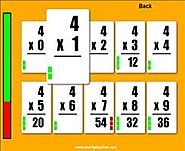Practice your multiplication facts in this fast paced flash card game. Multiplication will help you with multiplication and division of decimals by learning the basics first.

2

## Quick Flash Cards - Division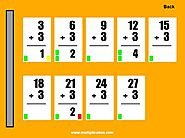Practice your division facts in this fast paced flash card game. Division will help you with multiplication and division of decimals by learning the basics first.

3

## Decimals Board Game for Tablets and iPads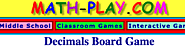Play this game with a partner to practice adding, subtracting, multiplying and dividing fractions.

4

## Flipgrid | Empower Every VoiceFlipgrid empowers you to express your mathematical thinking by explaining your process. You can share them with others and see their responses.

5

## Spy Guys - Multiplying and Dividing Decimals Game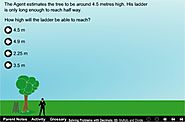Each task is based around solving problems with decimals. It might involve adding or subtracting decimals, estimating, or multiplication and division. Help spy guy finish his jobs by completely the tasks.

6

## IXL | Divide by decimals | 5th grade math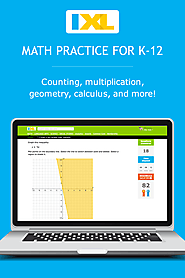Improve your math knowledge with free questions in "Divide by decimals" and thousands of other math skills.

7

## IXL | Multiply two decimals: products up to hundredths | 5th grade mathImprove your math knowledge with free questions in "Multiply two decimals: products up to hundredths" and thousands of other math skills.

8

## Multiplying DecimalsThis video is about Multiplying Decimals.

9

## Dividing DecimalsThis video is about Dividing Decimals.

10

## Boom Cards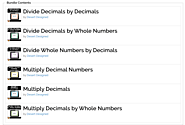You will practice multiplication and division with decimals with this set of Boom Cards.

Problems begin easy and as you work through the various decks, the problems increase in difficulty.

11

## How to divide decimals | Math, Decimals, Dividing, Dividing Decimals, long division, Middle School Math | ShowMe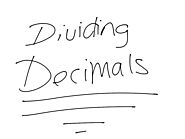step-by-step how to divide decimals

12

## 6-4 Multiplying Decimals with Area Models | Math, Elementary Math, 5th grade math, Decimals | ShowMe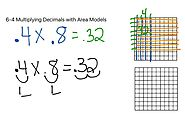step-by-step process about multiplying decimals with visuals

13

## Multiplying Decimals Song | How to Multiply Decimals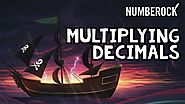14

## Kids Math: Multiplying and Dividing Decimals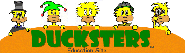Examples of how to multiply and divide decimals. How to line up and move the decimal points.

15

## Power Football - a game on Funbrain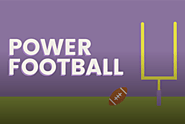Suitable for grades 4 - 6, Power Football lets you play football while solving math problems. Score a goal to win. Click on multiplication or division and then your level of difficulty.